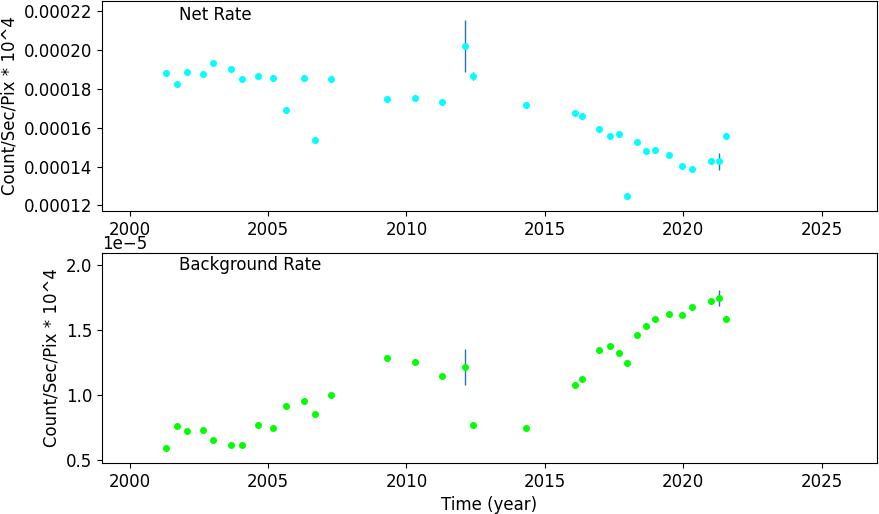## HRC S: Scaled Sum Amp Negative Range: 80 - 90Å

Hover the mouse over the data point on the plot below to see the information about the data point.
(Note: it may take a while to load the interactive plot.)

The fitted line on the popup window is a Gamma distribtuion:

g(x; k, θ) =
θk Γ(k)
xk-1 exp(-θ* x)
where k is the shape parameter and θ is the scale parameter.

### Count Rate: HRC S: Scaled Sum Amp Negative Range: 80 - 90